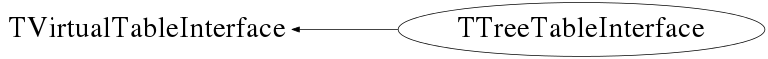# class TTreeTableInterface: public TVirtualTableInterface

```
TTreeTableInterface.

TTreeTableInterface is used to interface to data that is stored in a
TTree. When the interface is created, an expression can be
specified. This expression will define the columns to be shown.

A selection criterium can also be specified. A TEntryList will be
created and applied to the TTree using this criterium.
a new TEntryList to use can be specified using SetEntryList.
TGTable->Update() will need to be called to show the effects.

WARNING: Do not apply an entrylist to the tree in any other way than
through the interface, this will have undefined consequences.

Columns can be manipulated using the appropriate methods. A
TGTable->Update is always needed afterwards to make the table aware
of the changes.

```

## Function Members (Methods)

public:
 TTreeTableInterface(const TTreeTableInterface&) TTreeTableInterface(TTree* tree = 0, const char* varexp = 0, const char* selection = 0, Option_t* option = 0, Long64_t nentries = 0, Long64_t firstentry = 0) virtual ~TTreeTableInterface() virtual void AddColumn(const char* expression, UInt_t position) virtual void AddColumn(TTreeFormula* formula, UInt_t position) static TClass* Class() virtual const char* GetColumnHeader(UInt_t column) virtual TEntryList* GetEntryList() virtual UInt_t GetNColumns() virtual UInt_t GetNRows() virtual const char* GetRowHeader(UInt_t row) virtual Double_t GetValue(UInt_t row, UInt_t column) virtual const char* GetValueAsString(UInt_t row, UInt_t column) virtual TClass* IsA() const TTreeTableInterface& operator=(const TTreeTableInterface&) virtual void RemoveColumn(UInt_t position) virtual void SetEntryList(TEntryList* entrylist = 0) virtual void SetFormula(TTreeFormula* formula, UInt_t position) virtual void SetSelection(const char* selection) virtual void ShowMembers(TMemberInspector&) virtual void Streamer(TBuffer&) void StreamerNVirtual(TBuffer& ClassDef_StreamerNVirtual_b)
private:
 void InitEntries() void SetVariablesExpression(const char* varexp) void SyncFormulas()

## Data Members

private:
 TEntryList* fEntries Currently active entries Long64_t fEntry Present entry number in fTree. Long64_t fFirstEntry First entry. Bool_t fForceDim Force dimension. TList* fFormulas Array of TTreeFormulas to display values TList* fInput Used for fSelector. TTreeFormulaManager* fManager Coordinator for the formulas. UInt_t fNColumns Amount of columns in the data Long64_t fNEntries Number of entries in the tree. UInt_t fNRows Amount of rows in the data TTreeFormula* fSelect Selection condition TSelectorDraw* fSelector Selector TTree* fTree Data in a TTree

## Class Charts## Function documentation

TTreeTableInterface(TTree* tree = 0, const char* varexp = 0, const char* selection = 0, Option_t* option = 0, Long64_t nentries = 0, Long64_t firstentry = 0)
``` TTreeTableInterface constructor.
```

``` TTreeTableInterface destructor.
```
void SetVariablesExpression(const char* varexp)
``` Compile the variables expression from the given varexp.
```
void SetSelection(const char* selection)
``` Set the selection expression.
```
void SyncFormulas()
``` Sync the formulas using the TTreeFormulaManager.
```
void InitEntries()
``` Initialise the TEntryList with the entries that match the
selection criterium.
```
Double_t GetValue(UInt_t row, UInt_t column)
``` Return the value of row,column. If the position does not exist
or does not contain a number, 0 is returned.
```
const char * GetValueAsString(UInt_t row, UInt_t column)
``` Return the content of row,column as string to use in a
TGTableCell label.
```
``` Return a string to use as a label for rowheader at column.
```
``` Return a string to use as a label for columnheader at column.
```

``` Return the amount of column available.
```

``` Return the amount of rows in the Tree.
```
void AddColumn(const char* expression, UInt_t position)
``` Add column according ot expression at position,
TGTable->Update() is needed afterwards to apply the change to
the TGTable.
```
``` Add column with formula at position, TGTable->Update() is needed
afterwards to apply the change to the TGTable.
```
void RemoveColumn(UInt_t position)
``` Remove column at position, TGTable->Update() is needed
afterwards to apply the change to the TGTable.
```
void SetFormula(TTreeFormula* formula, UInt_t position)
``` Set the TTreeFormula of position to formula.
```
void SetEntryList(TEntryList* entrylist = 0)
``` Set the currently active entrylist.
```
TTreeTableInterface(TTree* tree = 0, const char* varexp = 0, const char* selection = 0, Option_t* option = 0, Long64_t nentries = 0, Long64_t firstentry = 0)

`{ return fEntries; }`